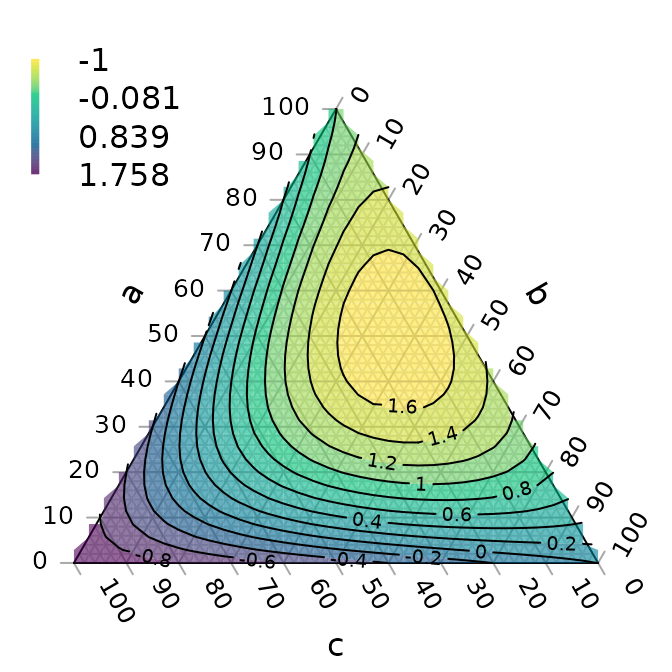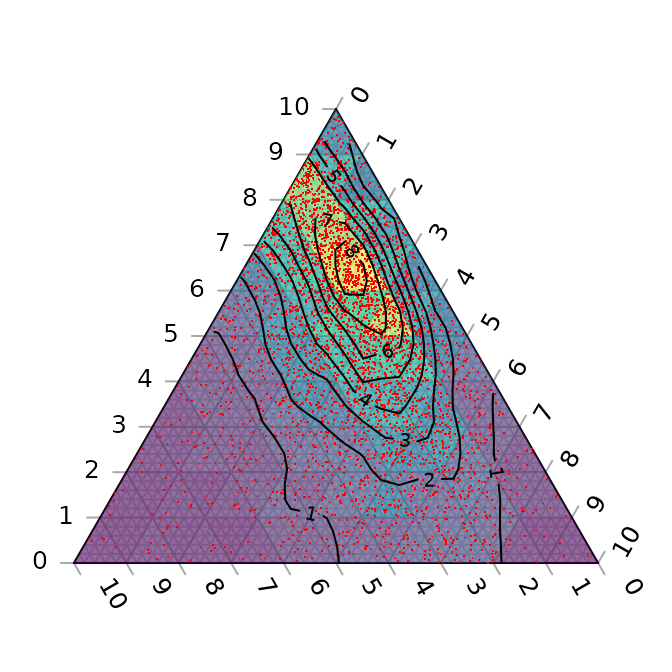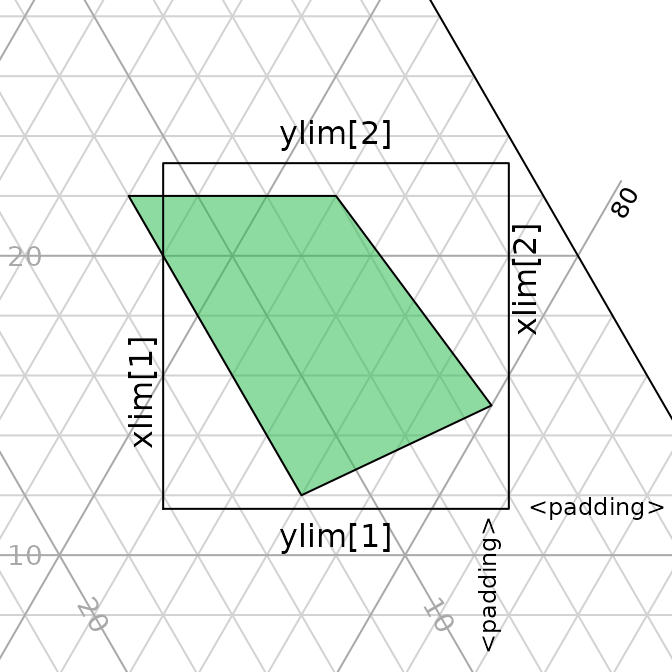“Ternary” is an R package to allow the creation of ternary plots in the standard R graphics environment. I hope that it proves simple to use.

For simple use cases, generate Ternary plots using the point-and-click Shiny app:

install.packages("Ternary")
Ternary::TernaryApp()

For greater control over your plots, use the full R implementation.

Install the package with:

install.packages("Ternary")

Or if you want the latest development version of the package:

if (!require("devtools")) install.packages("devtools")
install_github("ms609/Ternary")

Once the package is installed, load it into the current R session with

library("Ternary")

There are two stages to creating a ternary plot: first, rendering the plot, styled as you like it and pointing in any of the four compass directions; secondly, adding data.

## Create a blank plot

At its simplest, all you need to do is type

TernaryPlot()The following charts show which corners are which, under different orientations:

par(mfrow = c(2, 2), mar = rep(0.5, 4))
for (dir in c("up", "right", "down", "le")) {
TernaryPlot(point = dir, atip = "A", btip = "B", ctip = "C",
alab = "Aness", blab = "Bness", clab = "Cness")
TernaryText(list(A = c(10, 1, 1), B = c(1, 10, 1), C = c(1, 1, 10)),
labels = c("P1", "P2", "P3"),
col = cbPalette8, font = 2)
}## Plot two stylised plots side by side, and plot data

# Configure plotting area
par(mfrow = c(1, 2), mar = rep(0.3, 4))

# Initial plot
TernaryPlot(alab = "Redder \u2192", blab = "\u2190 Greener", clab = "Bluer \u2192",
lab.col = c("red", "darkgreen", "blue"),
main = "Colours", # Title
point = "right", lab.cex = 0.8, grid.minor.lines = 0,
grid.lty = "solid", col = rgb(0.9, 0.9, 0.9), grid.col = "white",
axis.col = rgb(0.6, 0.6, 0.6), ticks.col = rgb(0.6, 0.6, 0.6),
axis.rotate = FALSE,
# Colour the background:
cols <- TernaryPointValues(rgb)
ColourTernary(cols, spectrum = NULL)

# Add data points
data_points <- list(
R = c(255, 0, 0),
O = c(240, 180, 52),
Y = c(210, 222, 102),
G = c(111, 222, 16),
B = c(25, 160, 243),
I = c(92, 12, 243),
V = c(225, 24, 208)
)
AddToTernary(points, data_points, pch = 21, cex = 2.8,
bg = vapply(data_points,
function (x) rgb(x, x, x, 128,
maxColorValue = 255),
character(1))
)
AddToTernary(text, data_points, names(data_points), cex = 0.8, font = 2)
legend("bottomright",
legend = c("Red", "Orange", "Yellow", "Green"),
cex = 0.8, bty = "n", pch = 21, pt.cex = 1.8,
pt.bg = c(rgb(255,   0,   0, 128, NULL, 255),
rgb(240, 180,  52, 128, NULL, 255),
rgb(210, 222, 102, 128, NULL, 255),
rgb(111, 222,  16, 128, NULL, 255)),
)

###
# Next plot:
###
TernaryPlot("Steam", "Ice", "Water",
grid.lines = 5, grid.lty = "dotted",
grid.minor.lines = 1, grid.minor.lty = "dotted",
point = "West")

# Another way to add a title
title("Water phases", cex.main = 0.8)

# Add horizontal grid lines
HorizontalGrid()

# Define a polygon
middle_triangle <- matrix(c(
30, 40, 30,
30, 30, 40,
55, 20, 25
), ncol = 3, byrow = TRUE)

# Add polygon to plot
TernaryPolygon(middle_triangle, col = "#aaddfa", border = "grey")

# Connect corners of polygon to plot corners
TernaryLines(list(c(0, 100, 0), middle_triangle[1, ]), col = "grey")
TernaryLines(list(c(0, 0, 100), middle_triangle[2, ]), col = "grey")
TernaryLines(list(c(100, 0, 0), middle_triangle[3, ]), col = "grey")

# Add an arrow
TernaryArrows(c(20, 20, 60), c(30, 30, 40), length = 0.2, col = "darkblue")### Styling points

More sophisticated plots can be created, for example styling each point according to additional properties of the data, in a manner analogous to the standard plotting functions:

par(mar = rep(0.3, 4))

dat <- data.frame(sio2 = c(2, 4, 10, 20),
fe2o3 = c(5, 6, 7, 8),
al2o3 = c(12, 11, 10, 9),
grain_size = c(20, 16, 12, 8),
reflectance = c(80, 63, 61, 20))

spectrumBins <- 5
mySpectrum <- viridisLite::viridis(spectrumBins)
binnedReflectance <- cut(dat$reflectance, spectrumBins) dat_col <- mySpectrum[binnedReflectance] sizeBins <- 5 mySizes <- seq(0.5, 2.4, length.out = sizeBins) binnedSize <- cut(dat$grain_size, sizeBins)
dat_cex <- mySizes[binnedSize]

TernaryPlot(atip = expression(SiO),
btip = expression(paste(Fe, O, " (wt%)")),
ctip = expression(paste(Al, O))
)

TernaryPoints(dat[, c("sio2", "fe2o3", "al2o3")],
cex = dat_cex,
col = dat_col,
pch = 16
)

legend("topleft", col = dat_col, pch = 16,
legend = paste(binnedReflectance, "%"),
title = "Reflectance", bty = "n", cex = 0.8)

legend("topright", pt.cex = dat_cex, pch = 16,
legend = paste(binnedSize, "\u03bcm"),
title = "Grain size", bty = "n", cex = 0.8)See the “Points” tab of the shiny app for a point-and-click implementation.

### Cartesian coordinates

It is also possible to use cartesian coordinates to plot onto the graph.

By default, the plotting area is a 1×1 square.

par(mar = rep(0, 4)) # Reduce margins
TernaryPlot(point = "right", clockwise = FALSE)
cat("X range in this orientation:", TernaryXRange())
## X range in this orientation: -0.0669873 0.9330127
cat("Y range in this orientation:", TernaryYRange())
## Y range in this orientation: -0.5 0.5
arrows(x0 = 0.5, y0 = 0.4, x1 = sqrt(3) / 2, y1 = 0.4, length = 0.1,
col = cbPalette8)
text(x = mean(c(0.5, sqrt(3) / 2)), y = 0.4, "Increasing X", pos = 3,
col = cbPalette8)
text(x = 0.5, y = 0, "(0.5, 0)", col = cbPalette8)
text(x = 0.8, y = -0.5, "(0.8, -0.5)", col = cbPalette8)Note the anticlockwise axis labelling on this plot, obtained using clockwise = FALSE.

## Contours

A plot can be coloured and contoured according to the output of a mathematical expression:

par(mar = rep(0.2, 4))
TernaryPlot(alab = "a", blab = "b", clab = "c")

FunctionToContour <- function(a, b, c) {
a - c + (4 * a * b) + (27 * a * b * c)
}

# Compute and plot colour tiles
values <- TernaryPointValues(FunctionToContour, resolution = 24L)
ColourTernary(values)

# Add contour lines
TernaryContour(FunctionToContour, resolution = 36L)or by according to the density of points across the plot:

par(mar = rep(0.2, 4))
TernaryPlot(axis.labels = seq(0, 10, by = 1))

nPoints <- 4000L
coordinates <- cbind(abs(rnorm(nPoints, 2, 3)),
abs(rnorm(nPoints, 1, 1.5)),
abs(rnorm(nPoints, 1, 0.5)))

# Colour plot background
ColourTernary(TernaryDensity(coordinates, resolution = 10L))

TernaryPoints(coordinates, col = "red", pch = ".")

# Contour by point density
TernaryDensityContour(coordinates, resolution = 30L)The following image demonstrates the behaviour of the density estimates when points fall on boundaries of the triangular grid cells; text denotes the number of points within the cell, with cells straddling n cells contributing 1/n of a point to each cell straddled.## Magnification

Perhaps the action on a plot is constrained to a small region of ternary space. It’s possible to “zoom in” – i.e. magnify and crop the ternary plot to the region of interest.

To do this you can specify the x and y limits of the region of interest.

TernaryCoords might be useful in establishing the cartesian coordinates of a particular point in ternary space.
Ensure that dx = dy if you want an isometric plot.

my_corners <- list(c(22, 66, 12), c(22, 72, 6), c(15, 80, 5), c(12, 76, 12))
lapply(my_corners, TernaryCoords, direction = 1)
## []
##  0.2700000 0.1905256
##
## []
##  0.3300000 0.1905256
##
## []
##  0.3750000 0.1299038
##
## []
##  0.320000 0.103923

The padding parameter is added as a margin to each side of the region specified using xlim and ylim:

TernaryPlot(xlim = c(0.28, 0.38), ylim = c(0.1, 0.2), padding = 0.04)

# Annotate grid lines at user-specified points:
TernaryText(list(c(8, 72, 20), c(8, 82, 10)), c(20, 10), srt = -60, cex = 0.9,
col = "darkgrey")
TernaryText(list(c(10, 69, 21), c(20, 64, 16)), c(10, 20), srt = 0, cex = 0.9,
col = "darkgrey")

# Plot desired polygon
TernaryPolygon(my_corners, col = "#2cbe4e")

# Show xlim, ylim and padding, using cartesian coordinates
lines(c(0.28, 0.28, 0.38, 0.38, 0.28), c(0.1, 0.2, 0.2, 0.1, 0.1))
text(0.28, 0.15, "xlim", pos = 2, srt = 90)
text(0.38, 0.15, "xlim", pos = 4, srt = 90)
text(0.33, 0.1, "ylim", pos = 1)
text(0.33, 0.2, "ylim", pos = 3)
text(0.38, 0.1, "<padding>", pos = 4, cex = 0.75)
text(0.38, 0.1, "<padding> ", pos = 2, cex = 0.75, srt = 90)## Further tutorials

For further examples of usage, see the accompanying vignettes:

## Bugs and feature requests

I hope the package proves useful. If there”s anything it can”t do that you wish it could, please let me know by opening a Github issue.